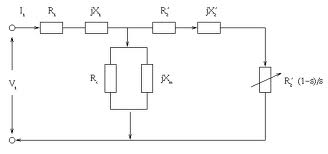## Equivalent Circuit Assignment Help

Assignment Help: >> Induction Motors - Equivalent Circuit

Equivalent Circuit:

We know that  E1  / E2 = a  and I2′ / I2  = 1 .

The impedance of the rotor circuit

2  = R2  + j S X 2

And   Z2  = R2  + j X 2  (at standstill)

2  = S E¯2/(R2  + j S X 2 )

Z 2′ = a2  R2  + j S a2   X 2

Z 2′ = R2′ + j S X 2

which gives

I ′ =   S E¯1/ (R2′ + j S X2 ′)    =    E¯1/(R′/S) + j X 2

The Eq. (51) refers the rotor circuit to the stator frequency f. In referring the rotor circuit to the stator frequency the reactance becomes constant ( X 2′ ) and the resistance becomes variable ( R2′ / S ) . The equivalent circuit of an induction motor is illustrated in Figure .If R2′ is separated from R2′ /S to represent the rotor copper loss as a separate quantity, the circuit model may be redrawn as Figure 5.16 where R2′ ((1/s)- 1) represents the mechanical output in electrical form.#### Assured A++ Grade

Get guaranteed satisfaction & time on delivery in every assignment order you paid with us! We ensure premium quality solution document along with free turntin report!

All rights reserved! Copyrights ©2019-2020 ExpertsMind IT Educational Pvt Ltd